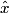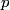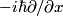## Solution to 1986 Problem 49

 Operators in quantum mechanics are in general obtained from classical functions by replacing$x$ by the operator$\hat{x}$ and$p$ with the operator$-i \hbar\partial/\partial x$. Therefore, answer (C) is correct.